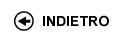Politecnico di Torino
04KWQLI, 04KWQJM, 04KWQLM, 04KWQLP, 04KWQLS, 04KWQLX, 04KWQLZ, 04KWQMA, 04KWQMB, 04KWQMC, 04KWQMH, 04KWQMK, 04KWQMO, 04KWQMQ, 04KWQNX, 04KWQOD, 04KWQPC, 04KWQPI, 04KWQPL
Mathematical analysis I
Corso di Laurea in Ingegneria Dell'Autoveicolo (Automotive Engineering) - Torino
Corso di Laurea in Ingegneria Meccanica (Mechanical Engineering) - Torino
Corso di Laurea in Ingegneria Informatica (Computer Engineering) - Torino
Espandi...
 Docente Qualifica Settore Lez Es Lab Tut Anni incarico Boieri Paolo 60 40 0 0 7 Boieri Paolo 60 40 0 0 7
 SSD CFU Attivita' formative Ambiti disciplinari MAT/05 10 A - Di base Formazione scientifica di base
Esclusioni:
16ACF
 Presentazione This course represents a bridge between high school and university. The main goal of the course is to provide the students with tools to follow the concatenation of simple logical arguments, and to introduce the fundamentals of differential and integral calculus for functions of one variable, with applications to ordinary differential equations of the first and the second order. Risultati di apprendimento attesi Ability to follow a chain of logical arguments. Knowledge of the fundamentals of differential and integral calculus for functions of one variable. Learning of an adequate computational ability. Prerequisiti / Conoscenze pregresse Numerical sets, equations and inequalities, analytic geometry and trigonometry. Elementary functions and their first properties. Programma Preliminaries: sets, operations with sets and logical symbols. Numerical sets, maxima, minima and extrema. The completeness property of real numbers and its consequences. Functions: surjectivity and injectivity; composition of functions, inverse functions. Functions of one real variable: elementary functions, monotone functions and inverse functions. (About 15 hours) Limits and continuity: limits of functions and sequences; continuity. Theorems on limits: uniqueness of the limit, sign-preserving property and local boundedness, comparison theorems. Limits of monotone functions. Algebra of limits. Indeterminate forms. Local comparison of functions. Landau symbols. Infinite and infinitesimal functions. Order of an infinity and of an infinitesimal, principal part with respect to a test function. Asymptotes. The number e. Fundamental trigonometric and exponential limits. Continuous functions over an interval: existence of zeros and of maxima and minima. (About 24 hours) Derivatives: geometrical and physical meaning. Computation of derivatives. Derivatives of elementary functions. Derivatives and continuity. Non-differentiability points, extremal and critical points. Fermat Theorem. Functions differentiable over an interval and fundamental theorems of differential calculus (Rolle and Lagrange) and their consequences. De L'H�pital rule. Taylor formula and fundamental Maclaurin expansions. Use of Taylor expansions in the local analysis of functions: comparison, extrema and convexity. Applications to the study of the graph of a function. (About 23 hours) Primitives and their computation; primitives of rational functions. Indefinite integral. The definite integral of a piecewise continuous function. Properties of the integral. Integral mean, the mean value theorem and the Fundamental Theorem of Calculus. Relations between definite integrals and primitives. Improper integrals: definitions and convergence tests. (About 21 hours) Complex numbers and differential equations: algebraic and trigonometric form of a complex number. Real and imaginary parts, modulus and argument. Roots of complex numbers; the Fundamental Theorem of Algebra. The exponential of a complex number and the Euler formula. Ordinary differential equations: the Cauchy problem. First order ordinary differential equations, linear or with separable variables. Second order linear differential equations with constant coefficients. (About 17 hours) Organizzazione dell'insegnamento 60 hours of theoretical classes and 40 hours of exercise classes. Testi richiesti o raccomandati: letture, dispense, altro materiale didattico C. Canuto, A. Tabacco. Mathematical Analysis I. Springer-Verlag UTX, 2015. Additional material will be provided by the teacher and will be available in the Internet personal page of the student. Criteri, regole e procedure per l'esame The exam consists of a test, a written part and, when required, of an oral part. The test (on a computer, one hour long) consists of 20 multiple-choice questions, also of theoretical type. Each question is worth one point, and the maximum achievable score is 20. If the test score is less than 12, then the exam is failed, otherwise the student proceeds with the written part of the exam (consisting of exercises and theoretical questions, one hour long). The written part is worth 13 points. If the score achieved is less than 5, then the exam is failed, otherwise the sum of the scores of the test and the written part is the final score of the exam, unless an oral part is required by the teacher (or by the student, provided that the final score is at least 18/30). In this case the final score will be based also on the oral part. Orario delle lezioni Statistiche superamento esami Programma definitivo per l'A.A.2016/17© Politecnico di Torino
Corso Duca degli Abruzzi, 24 - 10129 Torino, ITALY